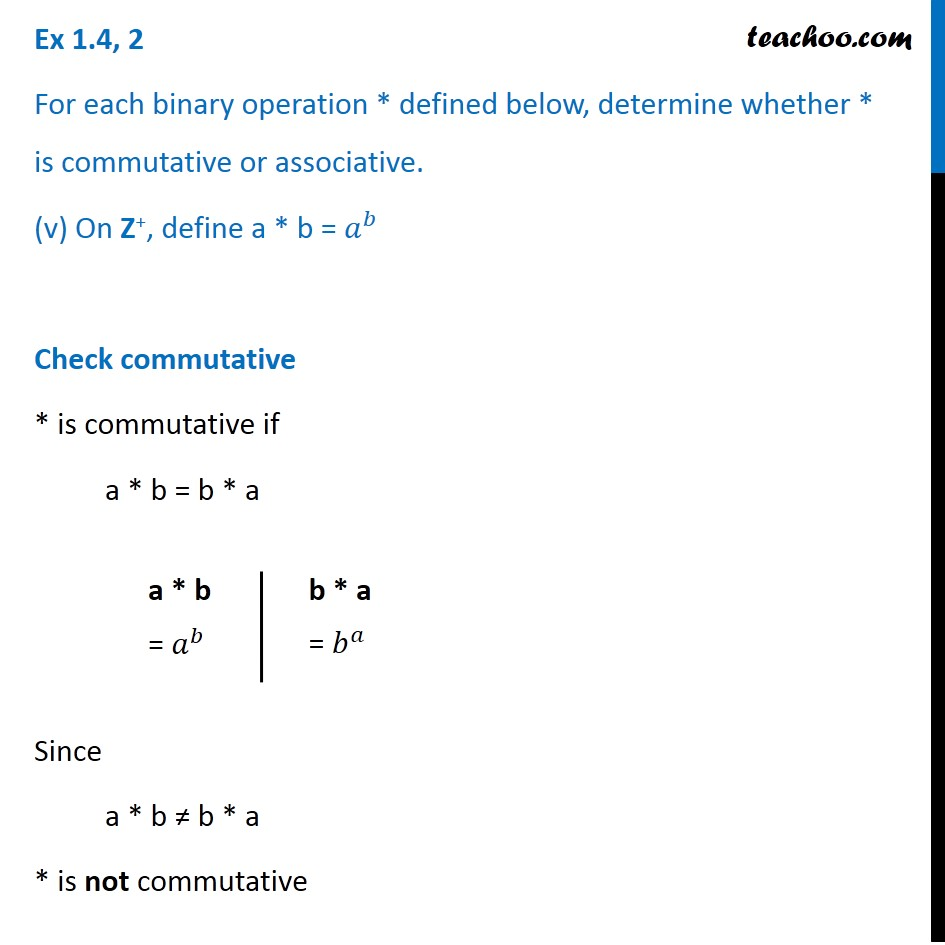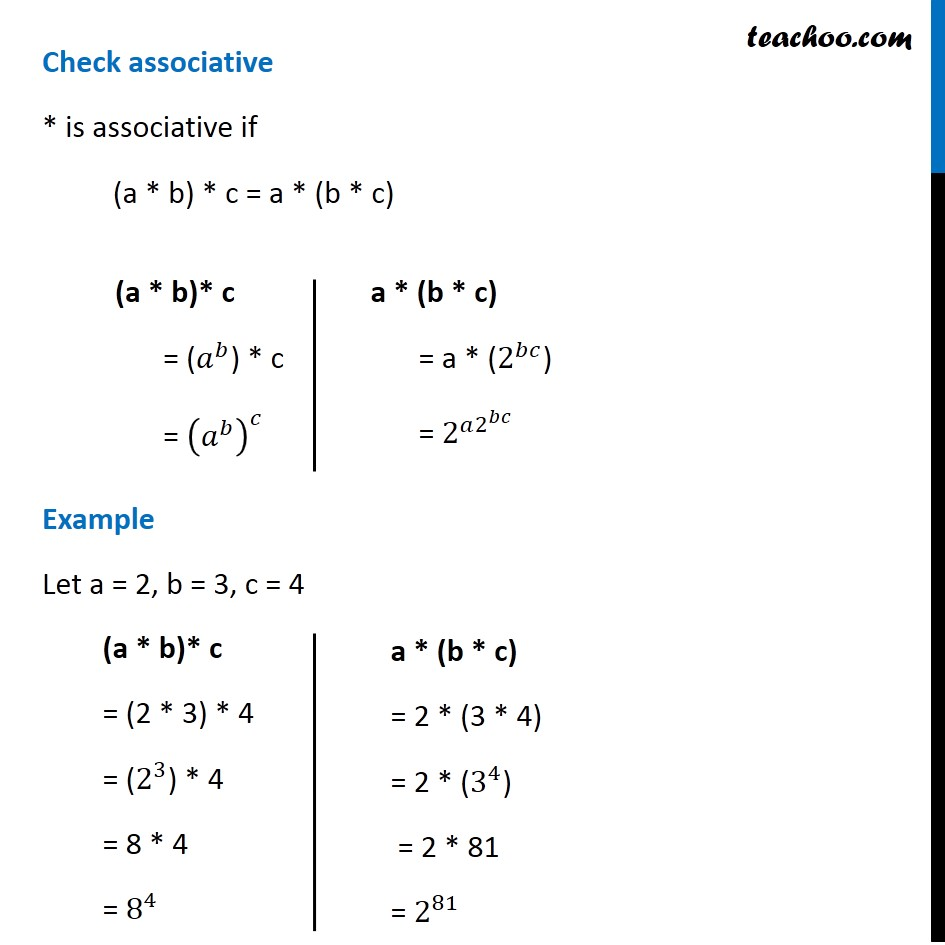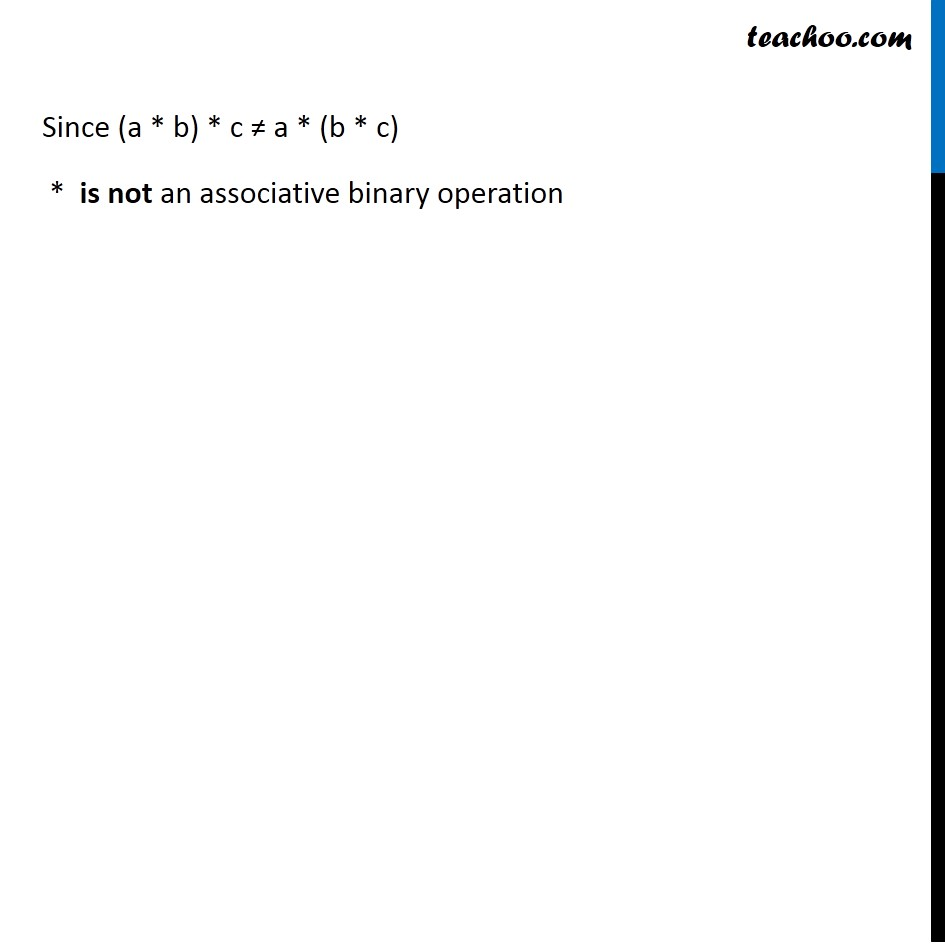1. Chapter 1 Class 12 Relation and Functions (Term 1)
2. Serial order wise
3. Ex 1.4

Transcript

Ex 1.4, 2 For each binary operation * defined below, determine whether * is commutative or associative. (v) On Z+, define a * b = 𝑎^𝑏 Check commutative * is commutative if a * b = b * a Since a * b ≠ b * a * is not commutative a * b = 𝑎^𝑏 b * a = 𝑏^𝑎 Check associative * is associative if (a * b) * c = a * (b * c) Example Let a = 2, b = 3, c = 4 (a * b)* c = (𝑎^𝑏) * c = (𝑎^𝑏 )^𝑐 a * (b * c) = a * (2^𝑏𝑐) = 2^(𝑎2^𝑏𝑐 ) (a * b)* c = (2 * 3) * 4 = (2^3) * 4 = 8 * 4 = 8^4 a * (b * c) = 2 * (3 * 4) = 2 * (3^4) = 2 * 81 = 2^81 Since (a * b) * c ≠ a * (b * c) * is not an associative binary operation